Custom SearchJOINT VARIATION A quantity VARIES JOINTLY as two or more quantities, if it equals a constant times their product. For example, ff x, y, and z are variables and k is a constant, x varies jointly as y and z, if x - kyz. Note that this is similar to direct variation, except that there are two variable factors and the constant with which to contend in the one number; whereas in direct variation, we had only one variable and the constant. The equality, x = kyz, is equivalent to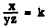If a quantity varies jointly as two or more other quantities, the ratio of the first quantity to the product of the other quantities is a constant. The formula for the area of a rectangle is an example of joint variation. If A is allowed to vary, rather than being constant as in the example used earlier in this chapter, then A varies jointly as L and W. When the formula is written for general use, it is not commonly expressed as A = kLW, although this is a mathematically correct form. Since the constant of proportionality in this case is 1, there is no practical need for expressing it. Using the formula A = LW, we make the following observations: If L = 5 and W = 3, then A = 3(5) = 15. If L = 5 and W = 4, then A = 4(5)= 20, and so on. Changes in the area of a rectangle depend on changes in either the length or the width or both. The area varies jointly as the length and the width. As a general example of joint variation, consider the expression a µ bc. Written as an equation, this becomes a = kbc. If the value of a is known for particular values of b and c, we can find the new value of a corresponding to changes in the values of b and c. For example, suppose that a is 12 when b is 3 and c is 2. What is the value of a when b is 4 and c is 5? Rewriting the proportion,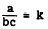ThusAlso,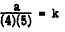Since quantities equal to the same quantity are equal to each other, we can set up the following proportion:Practice problems. Using k as the constant of proportionality, write equations that express the following statements: 1. 2 varies jointly as x and y. 2. S varies jointly as b times the square of r. 3. The length, W, of a radio wave varies jointly as the square root of the inductance, L, and the capacitance, C. Answers: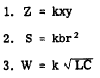COMBINED VARIATION The different types of variation can be combined. This is frequently the case in applied problems. The equation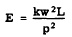is an example of combined variation and is read, "E varies jointly as L and the square of W, and inversely as the square of p." Likewise,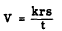is read, "V varies jointly as r and s and inversely as t."